• WORK
• TOOLS
• TRAINING
• VIEWS
• CONTACT

Views > Data visualization > Anscombe quartet

“Graphs are essential to good statistical analysis.”

F. J. Anscombe
English statistician

Six Sigma practitioners understood the importance of using graphical devices to monitor processes—instead of only relying on summary statistics.

Lets say we have a process producing tennis balls. Four samples of 20 balls each taken from the manufacturing process have an average of 67mm and a standard deviation of 0.45mm. The specifications are 65.5—68.5mm. The samples, along with summary statistics, are shown in the table below. Based on these results, how would you respond to such data? Should you intervene in the process or should you do nothing?

The charts below illustrate the shape of the data that produced the statistics provided above.

It’s clear from the charts that processes 2, 3 and 4 lack stability.

Most books on data visualization refer to the famous Anscombe Quartet to illustrate the importance of graphing data when performing statistical analysis. The Anscombe Quartet consists of four fictitious data sets, each consisting of 11 pairs of data as per the table below.

Although each of the four data sets yields the same regression model, when graphed, however, they depict completely different patterns as shown below.

# ANSCOMBE QUARTET

ANSCOMBE QUARTET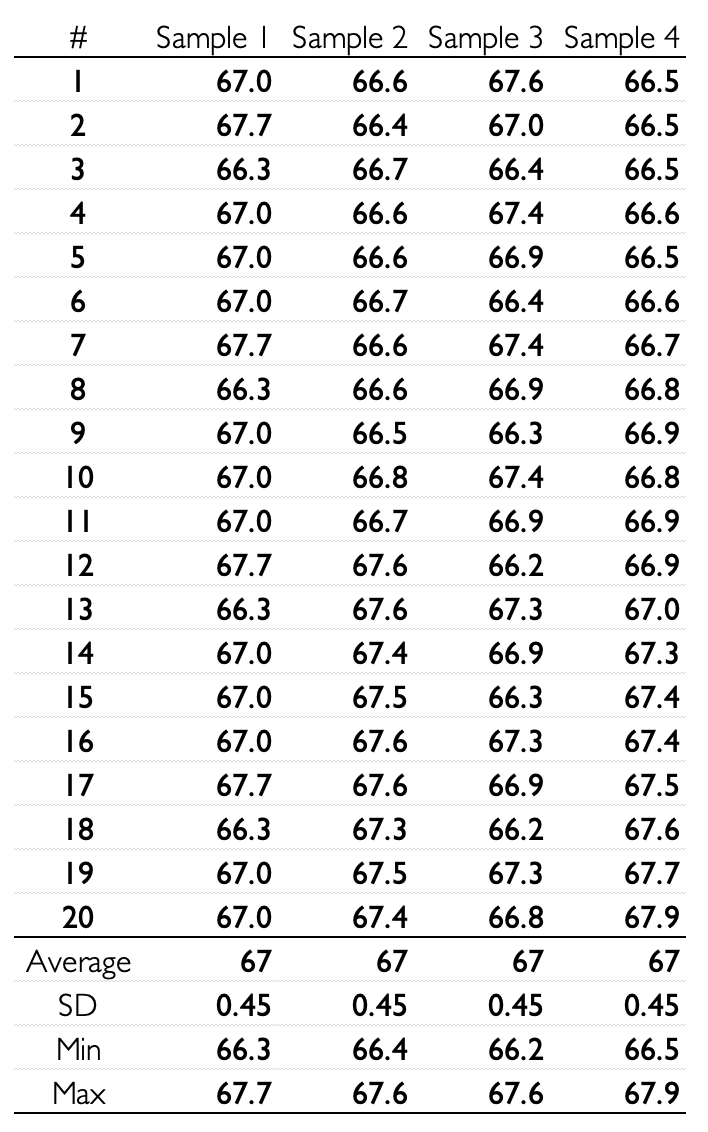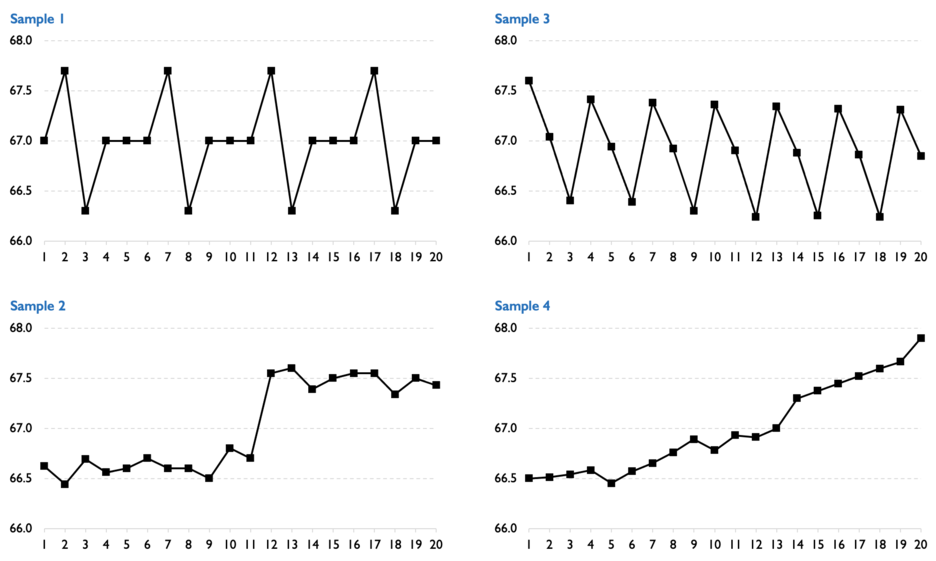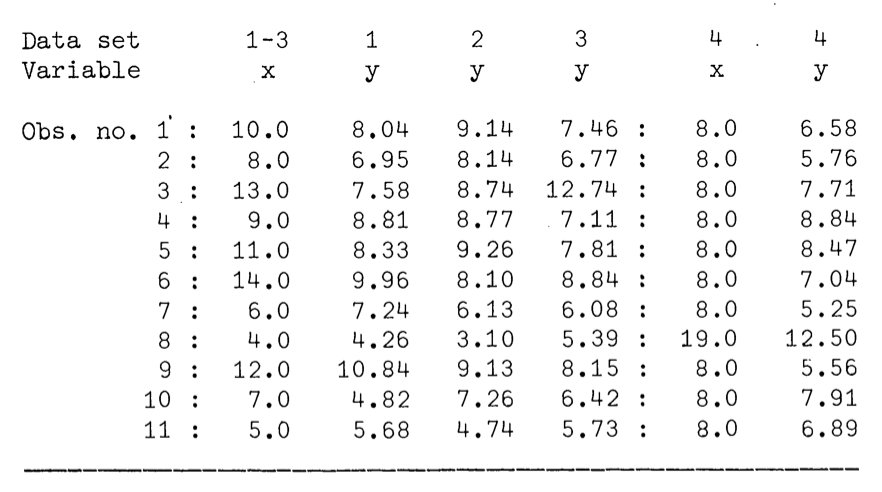Source: Graph in Statistical Analysis, F. J. Anscombe The American Statistician, Vol. 27, No. 1. (Feb., 1973), pp. 17-21.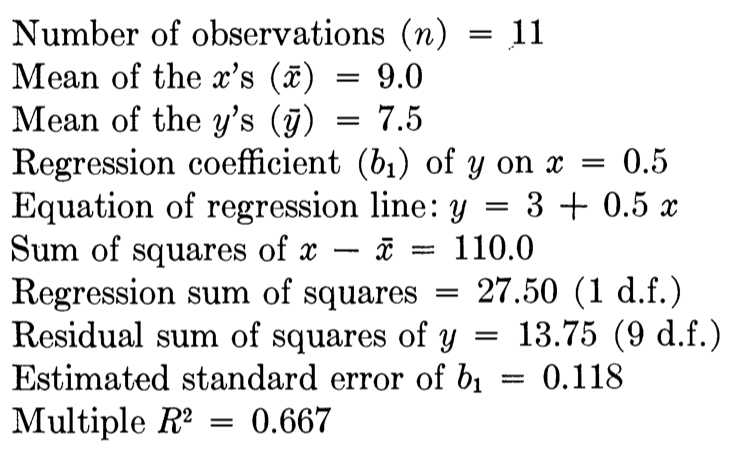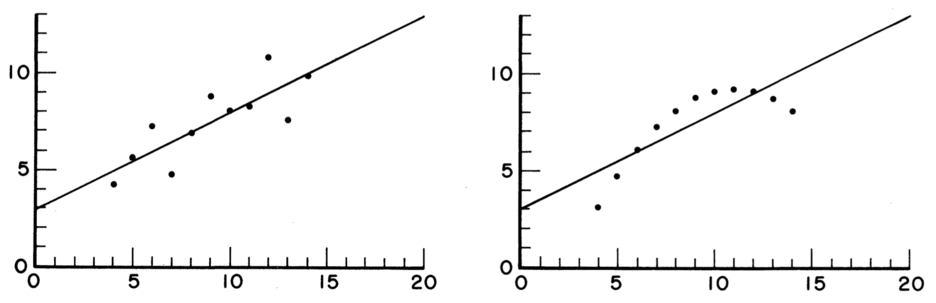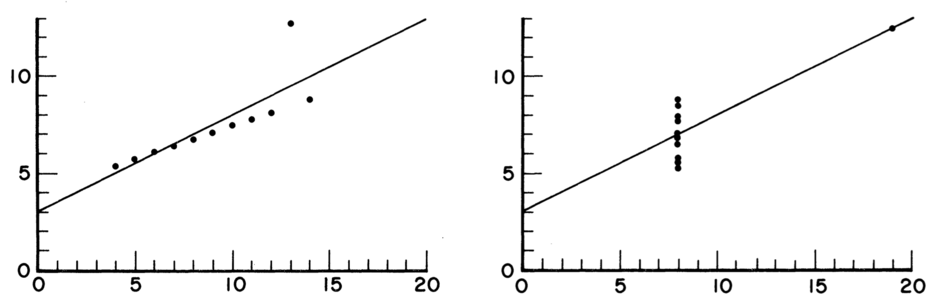Source: Graph in Statistical Analysis, F. J. Anscombe The American Statistician, Vol. 27, No. 1. (Feb., 1973), pp. 17-21.

Several algorithms for generating datasets with identical summary statistics while producing dissimilar graphics were created. Here are two such papers.

Same Stats, Different Graphs: Generating Datasets with Varied Appearance and Identical Statistics through Simulated Annealing by Justin Matejka and George Fitzmaurice.

Generating Data with Identical Statistics but Dissimilar Graphics by Chatterjee, S. and Firat, A.

Both of the above papers—including Wikipedia—claim that it is not clear how Anscombe came up with his data sets. Hence, my objective here is to create an Excel model that takes as an input a random data set and generate the three remaining data sets that look like Anscombe’s. That is, Quadratic, Linear and vertical.

Lets assume that we have a base data set consisting of N pairs of (xi,yi). We can calculate the statistical properties of this data set as follows: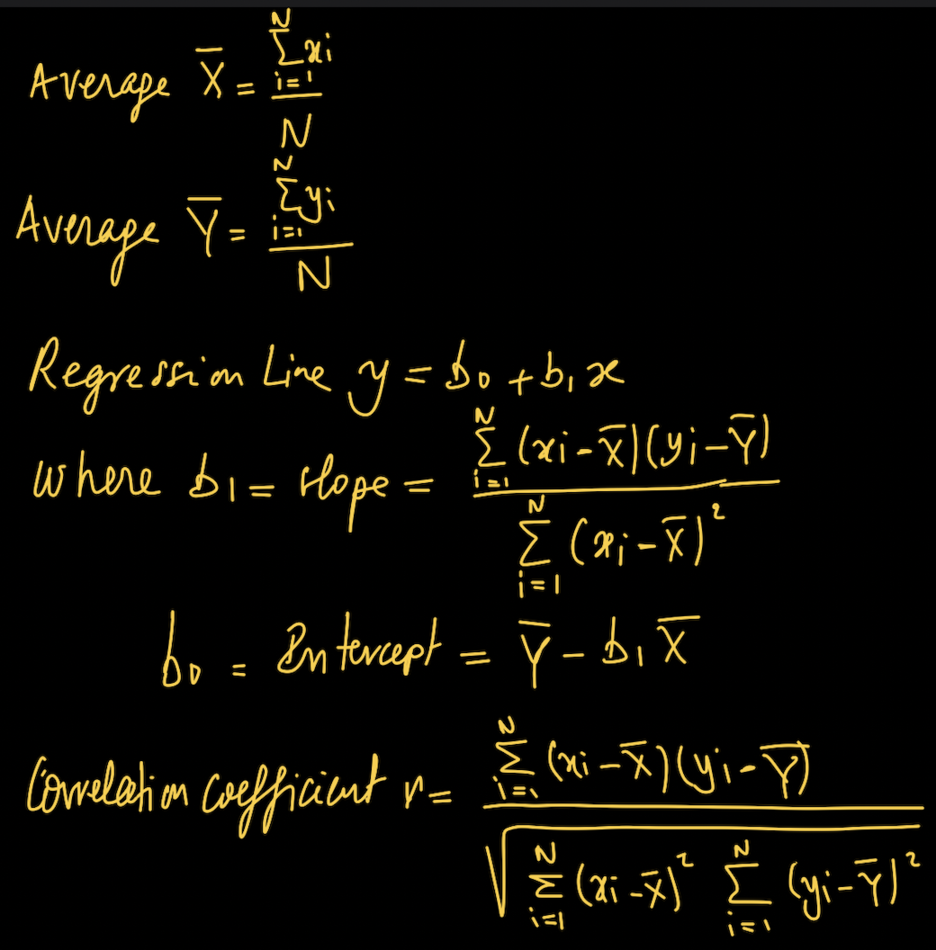To generate the quadratic model for set 2 we assume that the relation between y and x is as follows: y = mx2 + nx + p

I have three unknowns—m, n and p—therefore I need three independent equations to solve the problem. However, I’m going to create a set of two equations—(1) and (2) as below—with two unknowns m and n dependent on the third unknown p. Based on these two equations I’m going to use an iterative procedure that starts with say p = -1,000,000, evaluate m and n, calculate the correlation coefficient r and check the error with the base data set. If the error is above a certain specified epsilon, I adjust p and repeat the process till the model converges.

But before I do that note that as per Anscombe, datasets 1, 2 and 3 have the same xi.

The first equation is to use the average of yi as below:The second equation is to use the slope of the regression line b1 as below: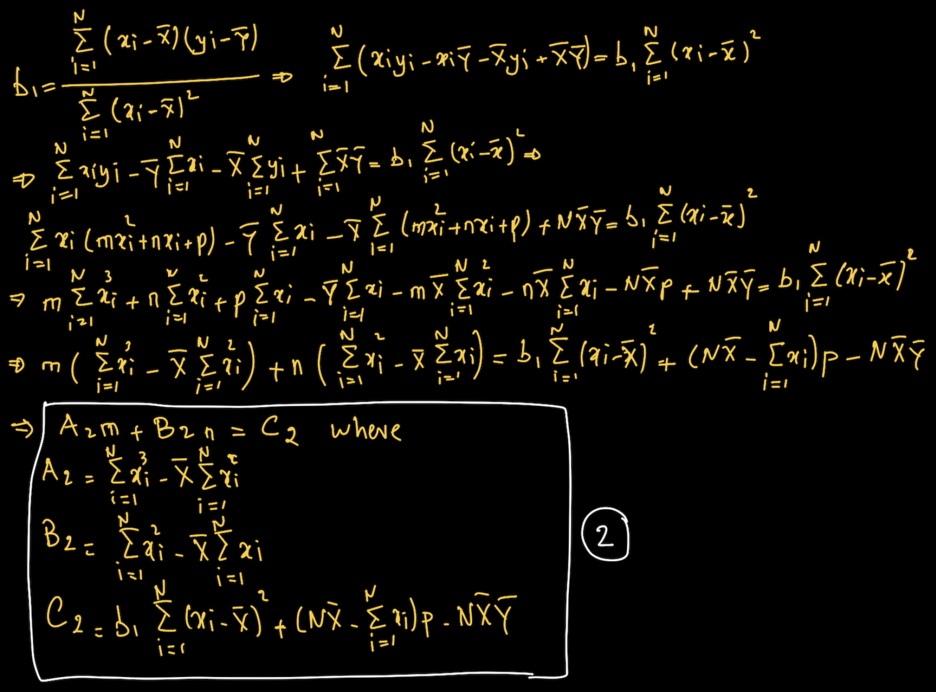LINEAR MODEL

I follow a similar procedure for the linear model of set 3. This time the relation between y and x is as follows: y = mx + n

Here too I have three unknowns—m and n defining the linear model along with yo, the vertical position of the outlier. Again I need three independent equations to solve the problem. However, I’m going to create a set of two equations—(1) and (2) as below—with two unknowns m and n dependent on the third unknown yo. Based on these two equations I’m going to use an iterative procedure that starts with say yo laying on the regression line, evaluate m and n, calculate the correlation coefficient r and check the error with the base data set. If the error is above a certain specified epsilon, I adjust yo and repeat the process till the model converges.

Again note that as per Anscombe, datasets 1, 2 and 3 have the same xi and the outlier is the data point with the second highest xi in the set (hence xo is known).

The first equation is to use the average of yi as below: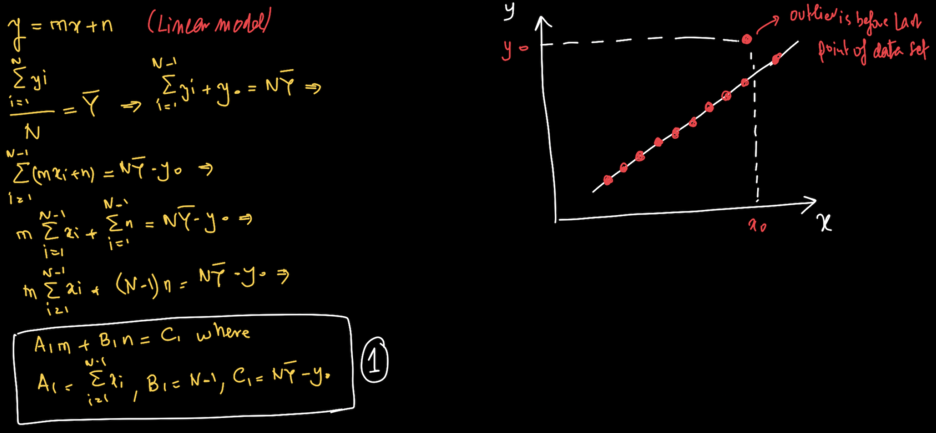The second equation is to use the slope of the regression line b1 as below: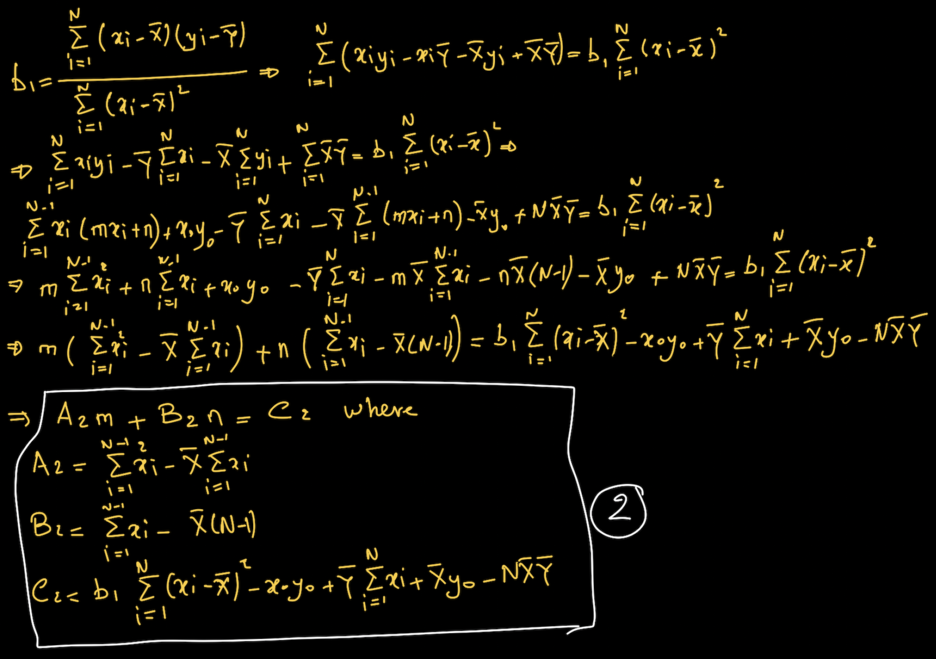VERTICAL LINE WITH OUTLIER

Here comes the difficult part. In this model all data points except one lie on a vertical line with abscissa x = m and the outlier is located at (xo,yo).

In order to calculate m and xo I use two equations, that is, the average of xi and their variance.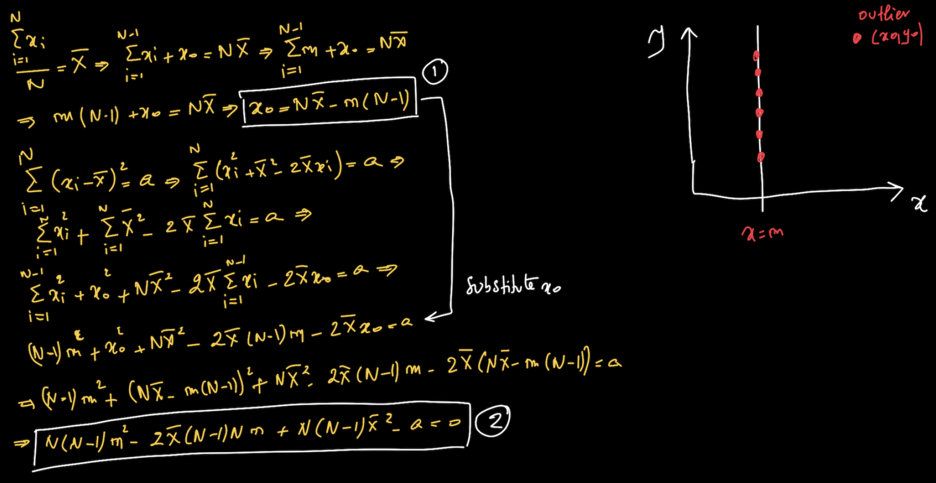I can evaluate yo by using the regression line at x = xo.To define the position of the remaining N – 1 data points along the line x = m, I start with the original data set yi, calculate the correlation coefficient r and check the error with the base data set. If the error is above a certain specified epsilon, I adjust all yi by a factor k and repeat the process till the model converges.

Here is the model solution for Anscombe’s original base set 1. You can see that set 2 and 3 are exactly the same as Anscombe’s. The third set is slightly different since there is an infinite way to lay the data points along the vertical axis. However the position of the vertical line x = 8, and the position of the outlier (19,12.5) are exactly the same.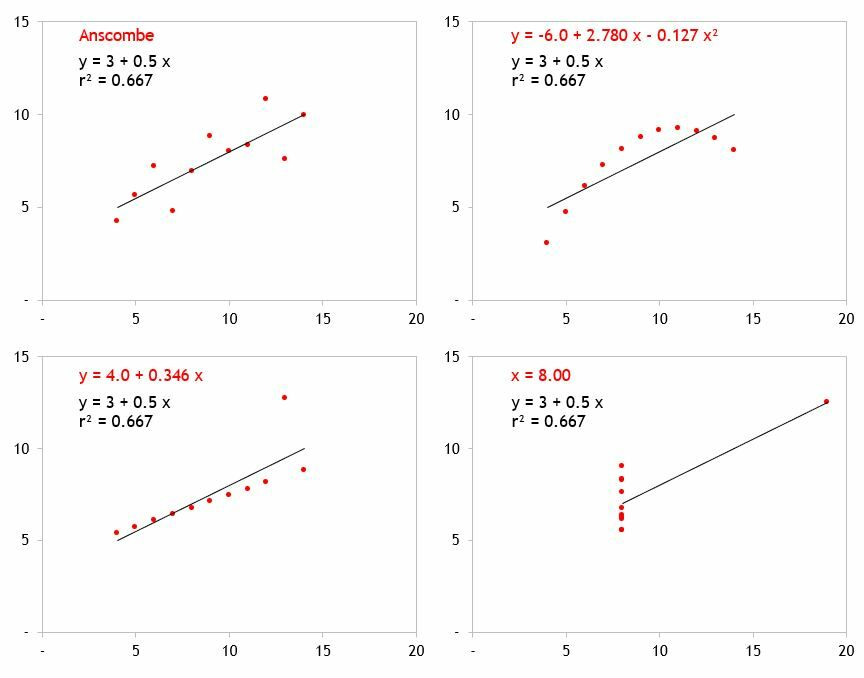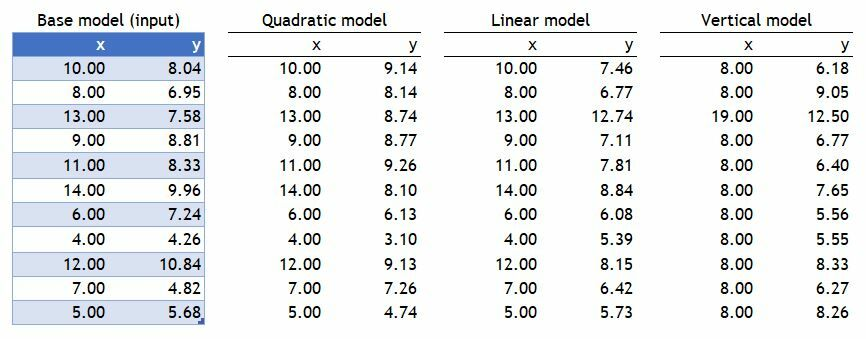Here below is the graphical display along with the regression line and r2.And here is the statistical model.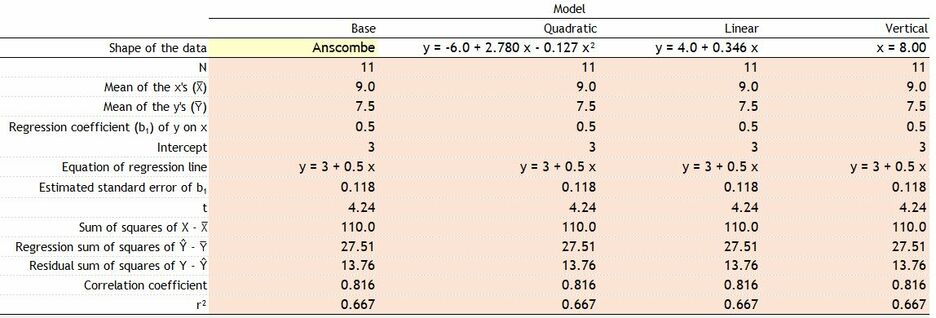And here are the model errors. As you can see the model converged with high precision.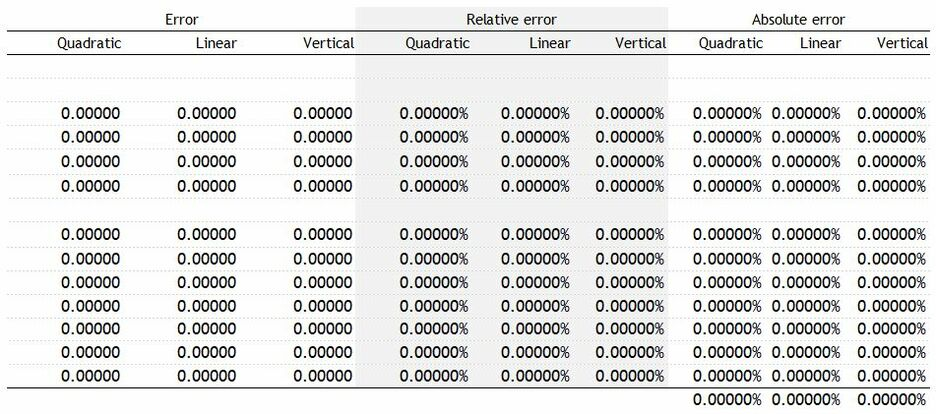Sometimes the model doesn’t converge to an exact solution. This may be due to different reasons:

1. A solution does not exist that fits the data.
2. The maximum number of iterations set in the model has been reached.
3. The algorithm used in the model cannot converge to a solution (this may be true for the x = m model).

Take this example. A Data set showing the correlation between disposable income in mUSD (x) in a certain geographic area and dishwasher sales in ‘000USD (y).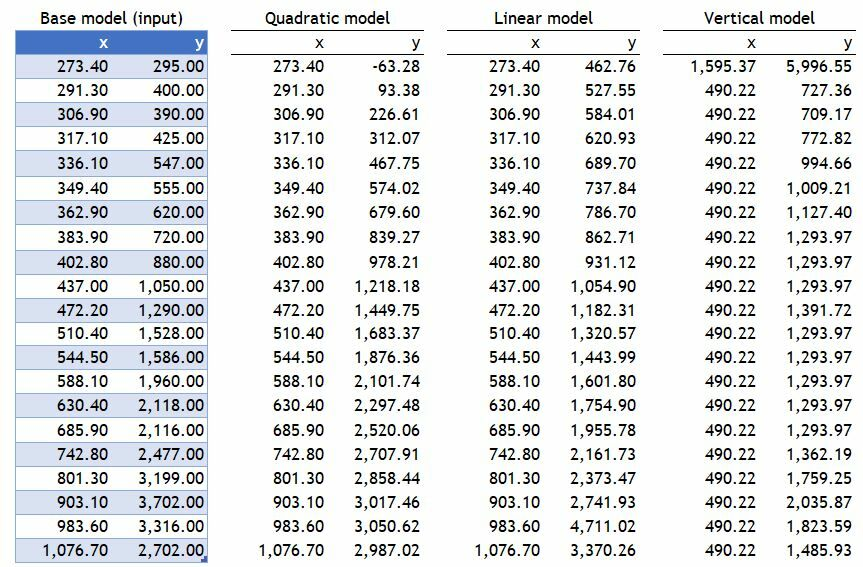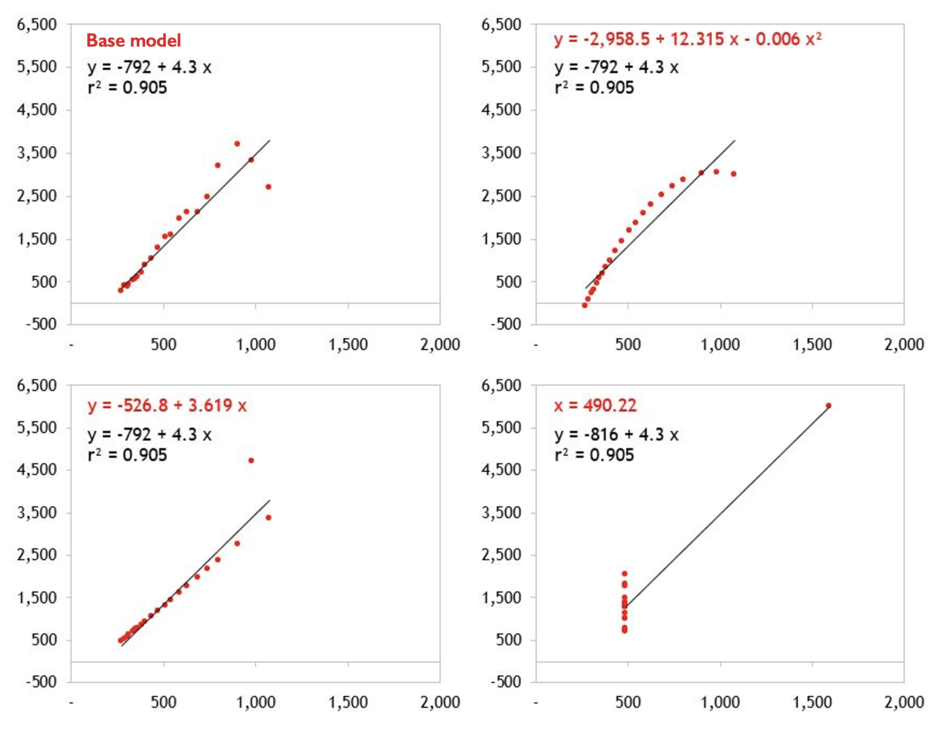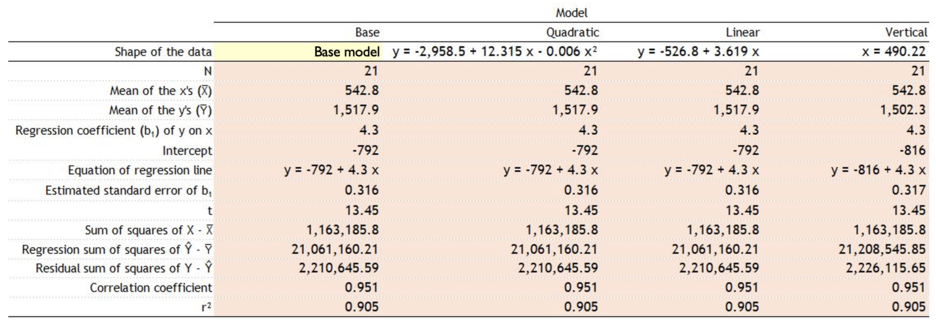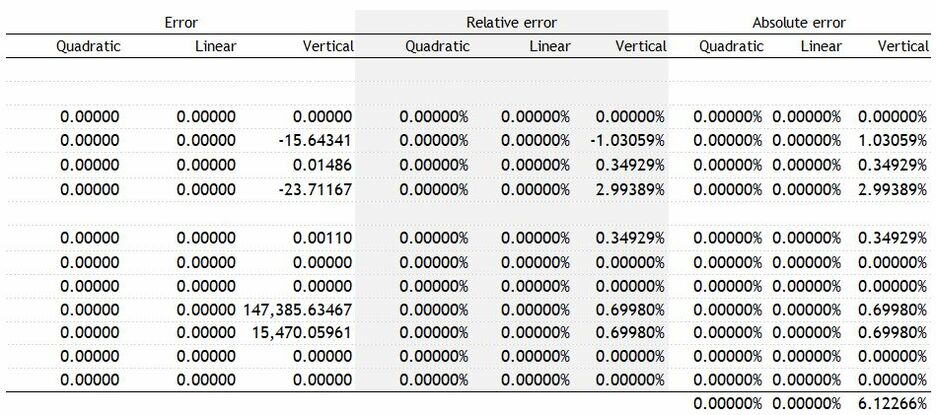You can see from the table above that the vertical model converged within an absolute total error of 6.1%. The highest error in the model is the intercept of the regression line where the result is 2.99% below that of the base case. The quadratic and linear models converged with high precision.

No analysis of Anscombe Quartet would be complete without testing for Alberto Cairo's Datasaurus.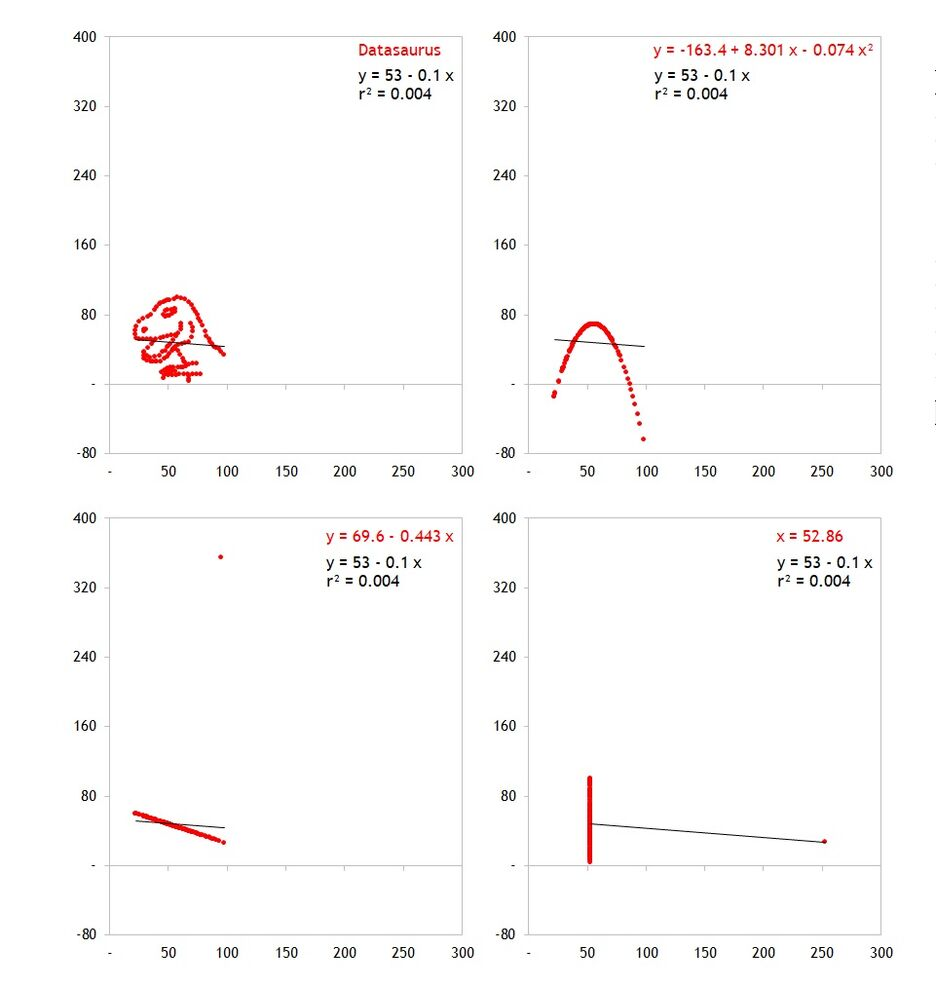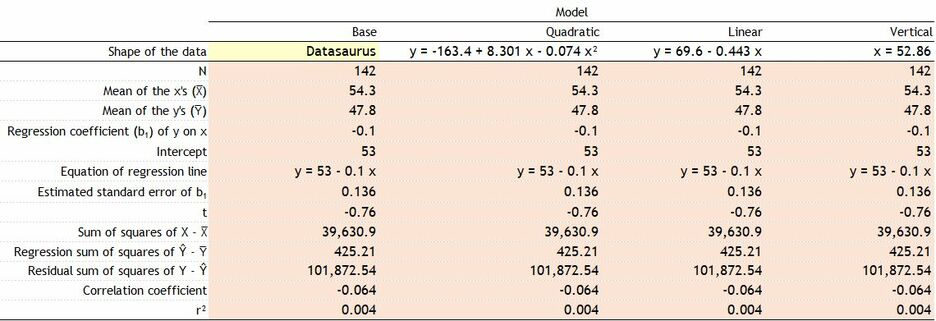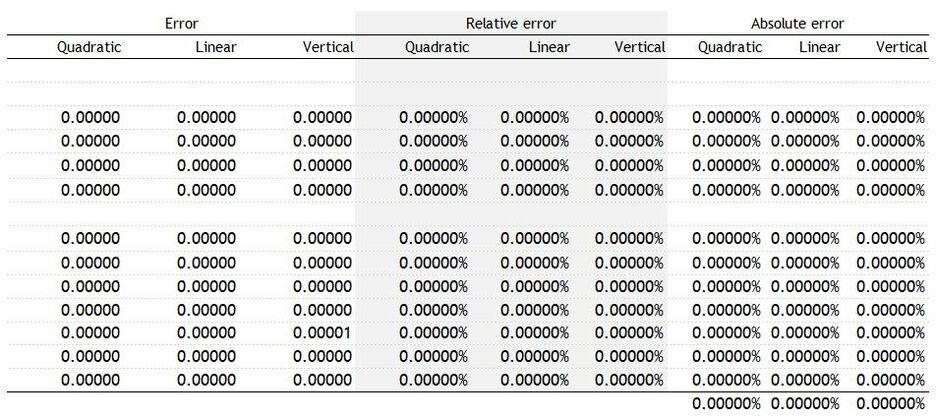File uses Dynamic Arrays available to Microsoft
Office 365 subscribers.